The number e as a limit

We have defined the natural logarithm as an integral, an 'area' under the rectangular hyperbola: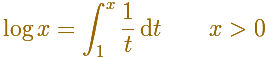Then we can define number e as the positive number at which log(e)=1:We could approximate its value using rectangles.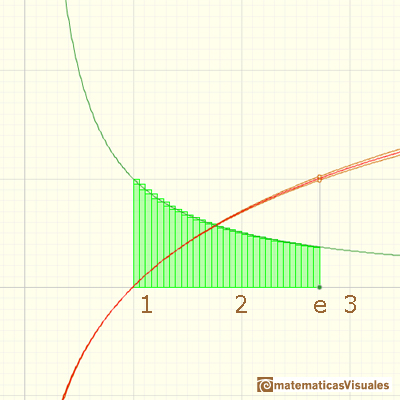But there is another popular definition of number e as a limit (related with compound interest):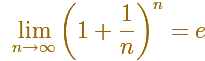These two definitions refer to the same number and we are going to see an intuitive approach to this.

To study the limit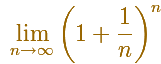you can consider, for each n, a series of rectangles whose bases are determined by the numbersIt is not difficult to see that there are n rectangles and that the area of each rectangles is 1/n (You need to make some calculations). Then the total area is 1.The last term of this seriesis an approximation by default of number e.

With more and more rectangles (bigger n) the approximation becomes better and better: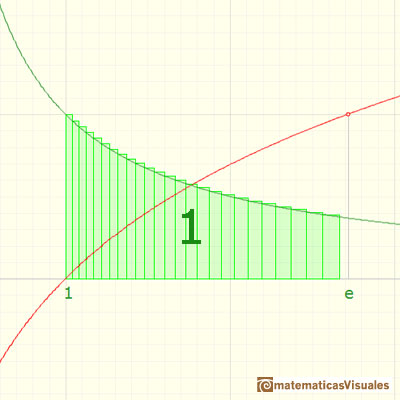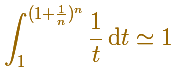Then we conclude thatUsing the applet you can see that the 'convergence' to number e is slow (to get a good approximations you need a big n).

There are better ways to calculate the value of e but this is a good approach to understand the idea of logarithm, integral and limit.By increasing the degree, Taylor polynomial approximates the exponential function more and more.

REFERENCES

A. I. Markushevich, Areas and Logarithms, D.C. Heath and Company, 1963.
Füsum Akman, Proofs Without Words Under the Magic Curve. The Mathematical Association of America (Classroom capsules).
Serge Lang, A First Course in Calculus, Third Edition, Addison-Wesley Publishing Company.
Tom M. Apostol, Calculus, Second Edition, John Willey and Sons, Inc.
Michael Spivak, Calculus, Third Edition, Publish-or-Perish, Inc.
Otto Toeplitz, The Calculus, a genetic approach, The University of Chicago Press, 1963.
Kenneth A. Ross, Elementary Analysis: The Theory of Calculus, Springer-Verlag New York Inc., 1980.We can study several properties of exponential functions, their derivatives and an introduction to the number e.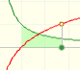Using the integral of the equilateral hyperbola we can define a new function that is the natural logarithm function.The natural logaritm can be defined using the integral of the rectangular hiperbola. In this page we are going to see an important property of this integral. Using this property you can justify that the logarithm of a product is the sum of the logarithms.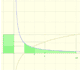The main property of a logarithm function is that the logarithm of a product is the sum of the logarithms of the individual factors.Different hyperbolas allow us to define different logarithms functions and their inversas, exponentials functions.Mercator published his famous series for the Logarithm Function in 1668. Euler discovered a practical series to calculate.By increasing the degree, Taylor polynomial approximates the exponential function more and more.The Complex Exponential Function extends the Real Exponential Function to the complex plane.The complex exponential function is periodic. His power series converges everywhere in the complex plane.The Fundamental Theorem of Calculus tell us that every continuous function has an antiderivative and shows how to construct one using the integral.The Second Fundamental Theorem of Calculus is a powerful tool for evaluating definite integral (if we know an antiderivative of the function).As an introduction to Piecewise Linear Functions we study linear functions restricted to an open interval: their graphs are like segments.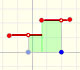A piecewise function is a function that is defined by several subfunctions. If each piece is a constant function then the piecewise function is called Piecewise constant function or Step function.A continuous piecewise linear function is defined by several segments or rays connected, without jumps between them.The integral of power functions was know by Cavalieri from n=1 to n=9. Fermat was able to solve this problem using geometric progressions.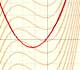If the derivative of F(x) is f(x), then we say that an indefinite integral of f(x) with respect to x is F(x). We also say that F is an antiderivative or a primitive function of f.The integral concept is associate to the concept of area. We began considering the area limited by the graph of a function and the x-axis between two vertical lines.Monotonic functions in a closed interval are integrable. In these cases we can bound the error we make when approximating the integral using rectangles.If we consider the lower limit of integration a as fixed and if we can calculate the integral for different values of the upper limit of integration b then we can define a new function: an indefinite integral of f.We can consider the polynomial function that passes through a series of points of the plane. This is an interpolation problem that is solved here using the Lagrange interpolating polynomial.